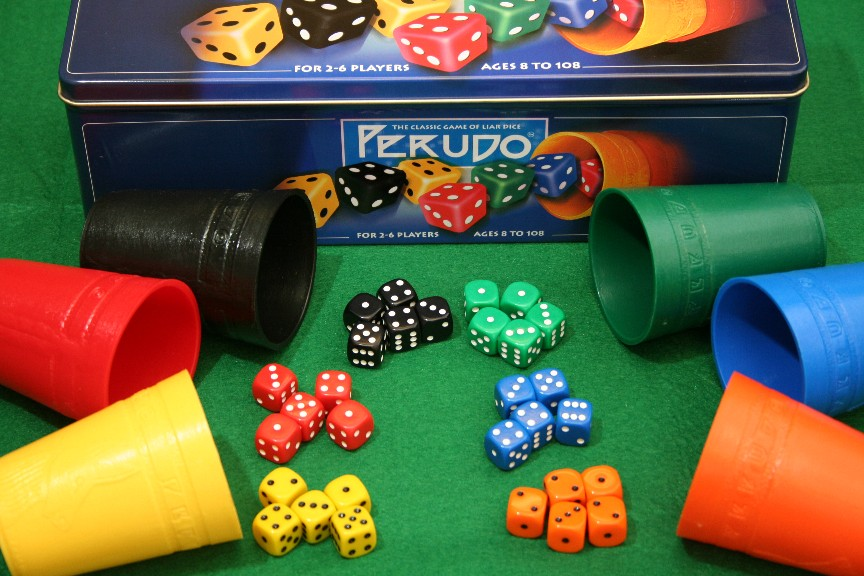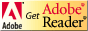In 2000, Hart and Mas-Colell introduced the important game-theoretic algorithm of regret matching.  Players reach equilibrium play by tracking regrets for past plays, making future plays proportional to positive cumulative regrets (i.e. how much they wished they had made the moves on average). The technique is not only simple and intuitive; it inspired the counterfactual regret minimization algorithm that has since formed the basis for bots dominating annual computer
poker competitions.

Since these algorithms are relatively recent, there are few curricular materials available to introduce students, researchers, and practitioners to regret-based algorithms. The tutorial PDF, suggested exercises, and sample code offered below represent a modest first step towards making such recent innovations more accessible.

 Summary An Introduction to Counterfactual Regret Minimization - Learn the game-theoretic regret-based techniques that spurred a revolution in competitive computer poker. Topics Game theory, regret, regret matching, counterfactual regret minimization (CFR), fixed strategy iteration counterfactual regret minimization (FSICFR), strategy purification, epsilon errors. Audience Advanced Artificial Intellegence students Difficulty Suitable for an upper-level undergraduate or graduate course with a focus on game theory, game AI, or other related topics.  A few weeks would be needed to complete all exercises. Strengths Students acquire a deep experiential knowledge of game-theoretic regret-based algorithms that leaves one close to the frontier of research in 2013.  For undergraduates, this would open doors to interesting undergraduate research projects suggested within.  For graduate students and researchers, these materials provide a fast means of grasping an important, emerging area of game theory. Weaknesses The presentation follows Knuth's literate programming style, and thus requires knowledge of the Java programming language. Dependencies Students must be mathematically literate and able to comprehend complex code and pseudocode. Students must also have advanced programming skills and be familiar with Java syntax and semantics. Variants Given that there are many simple and complex poker variants and bluffing dice games that have yet to receive research attention (see "Further Challenges" section), there are many possible follow-on projects and parallel examples that might be presented by the instructor.

# An Introduction to Counterfactual Regret Minimization

Index:## Overview

Using Java code examples in Donald Knuth's literate programming style, we will present example applications of the regret matching algorithm for normal form "one-shot" games, counterfactual regret minimization (CFR) for extensive form games, and fixed-strategy iteration counterfactual regret minimization (FSICFR).  We also briefly touch on strategy cleaning and how one might take maximal advantage of opponent error.  Throughout, the reader first sees an example application and is then invited to deepen understanding through application to additional challenge problems.

## Objectives

The object of this project is to give the student a deep, experiential understanding of game-theoretic regret-based algorithms through explanation, implementation examples, and implementation exercises

• Regret-Matching:
•• Explain basic definitions of game theory and the concept of regret.
• Present an outline of the regret-matching algorithm, along with a worked Java application example for the game of Rock-Paper-Scissors.
• Provide programming exercises for knowledge transfer.
• Counterfactual Regret Minimization (CFR):
• Explain the concept of counterfactual regret and the counterfactual regret minimization algorithm.
• Demonstrate its application to the simple game of Kuhn Poker.
• Specify a CFR application to the bluffing dice game of Dudo (restricted to 1-die-versus-1-die).
• Fixed-Strategy Iteration Counterfactual Regret Minimization (FSICFR):
• Explain the applicability of FSICFR to game graphs along with the reduction of CFR computational complexity from exponential to linear.
• Present FSICFR pseudocode and demonstrate its application in the context of our simple bluffing dice game, Liar Die.
• Present a challenge problem where one applies FSICFR to 1-die-versus-1-die Dudo with a claim memory of 3.
• Describe means of "cleaning" approximately optimal play policies in order to simplify and, in many cases, improve play.
• Describe an open research problem concerning how one might take maximal advantage of opponent error.

## Prerequisites

Students must be mathematically literate and able to comprehend complex code and pseudocode. Students must also have advanced programming skills and be familiar with Java syntax and semantics. Students who program in C++ should be able to follow most of the Java examples.

While not required, students with no prior exposure to game theory may wish to pair these materials with a brief primer on game theory online or in print (e.g. Leyton-Brown and Shoham's "Essentials of Game Theory", Morgan & Claypool, 2008).

Students are encouraged to play the games described in the materials to have a better appreciation of the decisions and a greater likelihood of discerning erroneous policy output.

## DescriptionThe projects are described in the file cfr.pdf.  You will need the free Adobe Acrobat Reader to view this file.Example code described in each project is available here:

This code was generated from the same source file that generated cfr.pdf using the literate programming tool noweb.

Students seeking more extended challenges will also find descriptions of related advanced projects in the last section of the document.

## Syllabus

Here is a sample syllabus used in (course omitted for review)

4/3: Essentials of Game Theory, Ch. 1, 2, Definition 3.2.1, Section 3.5; Handout: "Introduction to Counterfactual Regret Minimization", Sections 1-2; [Optional: S. Hart, A. Mas-Colell. A Simple Adaptive Procedure Leading to Correlated Equilibrium. Sections 2-3; H. Kuhn. Simplified Two-Person Poker.]
4/5: Handout: "Introduction to Counterfactual Regret Minimization", Section 3; T. Neller, S. Hnath.  Approximating Optimal Dudo Play with Fixed-Strategy Iteration Counterfactual Regret Minimization, through Section 2  [Optional: M. Zinkevich et al. Regret Minimization in Games with Incomplete Information.]
4/10: Handout: "Introduction to Counterfactual Regret Minimization", Section 4; T. Neller, S. Hnath.  Approximating Optimal Dudo Play with Fixed-Strategy Iteration Counterfactual Regret Minimization, remaining sections.
4/12: Handout "Introduction to Counterfactual Regret Minimization", Section 5
4/17: Handout "Introduction to Counterfactual Regret Minimization", Section 6

Assignments:

1. Regret - Rock-Paper-Scissors (Thursday 4/5): Read Section 1 of CFR handout in order to learn about game theoretic definitions, regret, regret matching and minimization.  Using the Rock-Paper-Scissors worked example of one-sided regret-matching, complete the two-sided regret-matching RPS Equilibrium, exercise 2.5.
2. Counter Factual Regret Minimization (CFR) - 1-die-versus-1-die Dudo  (Thursday 4/12): Read Section 2 of CFR handout in order to learn about CFR.  Using the Kuhn Poker worked example as a model, complete the 1-die-versus-1-die Dudo exercise of subsection 2.5
3. Error modeling (Thursday 4/19): - Read sections 3-5 of CFR handout in order to learn about cleaning results, FSICFR, and epsilon error.  Modify the Liar Die FSICFR worked example of subsection 4.3 so as to have clean results that maximally exploit potential opponent errors.